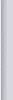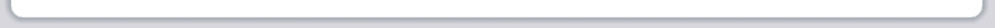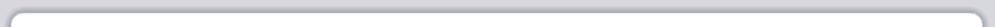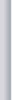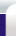Corporate Group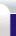C.I.S.TC.I.R.TMANAGEMENTPHARMACYPhoto GalleryDownloadsRecruitmentNews Coverage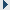1st Year2nd Year3rd Year4th YearCommon For All
1st Year
 Downloads/ View: 1. Maths Formulas List 11. Maths Formulas List 21. Maths Formulas List 31. Maths Formulas List 41. Maths Formulas List 51. Maths Formulas List 61. Maths Formulas List 71. BEEE Video Solution Part 11. BEEE Video Solution Part 21. BE- 1st Year RGPV Papers 2. EX-104 RGPV Exam Solution 3. BCE Practical Index- (BE-205) 4. Maths Assignment- (BE-102) 5. Maths Tutorial- (BE-102)